Magn. Reson., 1, 315–330, 2020
https://doi.org/10.5194/mr-1-315-2020
Magn. Reson., 1, 315–330, 2020
https://doi.org/10.5194/mr-1-315-2020

Research article 17 Dec 2020

Research article | 17 Dec 2020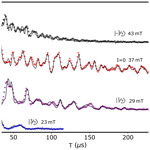# Hyperfine spectroscopy in a quantum-limited spectrometer

Hyperfine spectroscopy in a quantum-limited spectrometer
Sebastian Probst1, Gengli Zhang2, Miloš Rančić1, Vishal Ranjan1, Marianne Le Dantec1, Zhonghan Zhang3, Bartolo Albanese1, Andrin Doll4, Ren Bao Liu2, John Morton5, Thierry Chanelière6, Philippe Goldner3, Denis Vion1, Daniel Esteve1, and Patrice Bertet1 Sebastian Probst et al.
• 1Quantronics group, SPEC, CEA, CNRS, Université Paris-Saclay, CEA Saclay, 91191 Gif-sur-Yvette CEDEX, France
• 2Department of Physics and The Hong Kong Institute of Quantum Information Science and Technology, The Chinese University of Hong Kong, Shatin, New Territories, Hong Kong, China
• 3Chimie ParisTech, PSL University, CNRS, Institut de Recherche de Chimie Paris, 75005 Paris, France
• 4Laboratory of nanomagnetism and oxides, SPEC, CEA, CNRS, Université Paris-Saclay, CEA Saclay, 91191 Gif-sur-Yvette CEDEX, France
• 5London Centre for Nanotechnology, University College London, London WC1H 0AH, UK
• 6Univ. Grenoble Alpes, CNRS, Grenoble INP, Institut Néel, 38000 Grenoble, France

Correspondence: Patrice Bertet (patrice.bertet@cea.fr)

Abstract

We report measurements of electron-spin-echo envelope modulation (ESEEM) performed at millikelvin temperatures in a custom-built high-sensitivity spectrometer based on superconducting micro-resonators. The high quality factor and small mode volume (down to 0.2 pL) of the resonator allow us to probe a small number of spins, down to 5×102. We measure two-pulse ESEEM on two systems: erbium ions coupled to 183W nuclei in a natural-abundance CaWO4 crystal and bismuth donors coupled to residual 29Si nuclei in a silicon substrate that was isotopically enriched in the 28Si isotope. We also measure three- and five-pulse ESEEM for the bismuth donors in silicon. Quantitative agreement is obtained for both the hyperfine coupling strength of proximal nuclei and the nuclear-spin concentration.

Share
1 Introduction

Electron paramagnetic resonance (EPR) spectroscopy provides a set of versatile tools to study the magnetic environment of unpaired electron spins . EPR spectrometers rely on the inductive detection of the spin signal by a three-dimensional microwave resonator tuned to the spin Larmor frequency. While concentration sensitivity is the main concern for dilute samples available in macroscopic volumes , there are also cases in which the absolute spin detection sensitivity matters, motivating research towards alternative detection methods to measure smaller and smaller numbers of spins. Electrical , optical , and scanning-probe-based detection methods of magnetic resonance have reached sufficient sensitivity to detect individual electron spins.

In parallel, recent results have shown that the inductive detection method can also be pushed to much higher absolute sensitivity than previously achieved, using planar micro-resonators and micro-helices . Superconducting resonators are particularly useful in that context since they combine low mode volume and narrow linewidth κ. Inductive-detection spectrometers relying on a superconducting planar micro-resonator combined with a Josephson parametric amplifier (JPA), cooled down to millikelvin temperatures , have achieved a sensitivity of 10 spin$/\sqrt{\mathrm{Hz}}$ for detecting Hahn echoes emitted by donors in silicon . A particular feature of these quantum-limited spectrometers is that quantum fluctuations of the microwave field play an important role. First, the system output noise is governed by these quantum fluctuations, with negligible thermal noise contribution. Second, quantum fluctuations also impact spin dynamics by triggering spontaneous emission of microwave photons at a rate ${\mathrm{\Gamma }}_{\mathrm{P}}=\mathrm{4}{g}^{\mathrm{2}}/\mathit{\kappa }$, g being the spin–photon coupling . This Purcell effect forbids T1 from becoming prohibitively long since it is at most equal to ${\mathrm{\Gamma }}_{\mathrm{P}}^{-\mathrm{1}}$, making spin detection with a reasonable repetition rate possible even at the lowest temperatures.

Hahn echoes are the simplest pulse sequence used in EPR spectroscopy, useful to determine the electron-spin density as well as the spin Hamiltonian parameters and their distribution. The richness of EPR comes from the ability to characterize the local magnetic environment of the electron spins, often consisting of a set of nuclear spins or of other electron spins. For that, hyperfine spectroscopy is required, which uses more elaborate pulse sequences and requires larger detection bandwidth. Previous hyperfine spectroscopy measurements with superconducting micro-resonators include the electron–nuclear double resonance detection of donors in silicon and the electron-spin-echo envelope modulation (ESEEM) of erbium ions by the nuclear spin of yttrium in a Y2SiO5 crystal .

Here, we demonstrate that hyperfine spectroscopy is compatible with quantum-limited EPR spectroscopy despite its additional requirements in terms of pulse complexity and bandwidth, by measuring ESEEM in two model electron-spin systems. We measure the ESEEM of erbium ions coupled to 183W nuclei in a scheelite crystal (CaWO4) with a simple two-pulse sequence and get quantitative agreement with a simple dipolar interaction model. We also measure the ESEEM of bismuth donors in silicon caused by 29Si nuclei using two-, three-, and five-pulse sequences . Compared to other ESEEM measurements on donors in silicon , ours are performed in an isotopically purified sample having a 100 times lower concentration in 29Si (500 ppm) than natural abundance. As a result, the dominant hyperfine interactions in the ESEEM signal are very low (on the order of 100 Hz) and have to be detected at low magnetic fields (around 0.1 mT). These results bring quantum-limited EPR spectroscopy one step closer to real-world applications.

2 ESEEM spectroscopy: theory

## 2.1 Phenomenology

We start by briefly discussing the ESEEM phenomenon. Consider an ensemble of electron spins placed in a magnetic field B0. The spin ensemble linewidth Γ is broadened by a variety of mechanisms: spatial inhomogeneity of the applied field B0, local magnetic fields generated by magnetic impurities throughout the sample, and spatially inhomogeneous strain or electric fields. One prominent way to mitigate the effect of this inhomogeneous broadening is the spin-echo sequence (also called Hahn echo, or two-pulse echo). It consists of a π∕2 pulse at time t=0 and a π pulse after a delay τ (see Fig. 1a). This π pulse reverses the evolution of the phase of the precessing magnetic dipoles, which leads at a later time 2τ to their refocusing and the emission of a microwave pulse (the echo) of amplitude V2p(τ).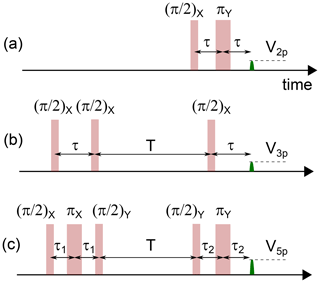Figure 1Sequences used for two-pulse (a), three-pulse (b), and five-pulse (c) ESEEM measurements.

Download

In general, V2p(τ) decays monotonically; it can however also display oscillations. Such ESEEM was first observed by and for Ce3+ ions in a CaWO4 crystal and was interpreted as being caused by the dipolar interaction of the electronic spin of the Ce3+ ions with the 183W nuclear spins of the crystal. The oscillation frequencies appearing in the ESEEM pattern are related to the nuclear-spin Larmor frequencies and to their coupling to the electron spin. As such, ESEEM measurements provide spectroscopic information on the nature of the nuclear-spin bath and its density, and ESEEM spectroscopy has become an essential tool in advanced EPR . ESEEM has also been observed for individual spins measured optically, in particular for individual nitrogen vacancy (NV) centers in diamond coupled to a bath of 13C nuclear spins . A more complete theory of ESEEM is presented in Mims (1972). Our goal here is to provide a simple picture of the physics involved, as well as to introduce useful formulas and notations.

## 2.2 Two-spin 1∕2 model

We follow the analysis in of the model case depicted in Fig. 2a. An electron spin of $S=\mathrm{1}/\mathrm{2}$, with an isotropic g tensor, is coupled to a proximal nuclear spin of $I=\mathrm{1}/\mathrm{2}$. Both are subject to a magnetic field B0 applied along z. The system Hamiltonian is

$\begin{array}{}\text{(1)}& {\mathbf{H}}_{\mathrm{0}}={\mathbf{H}}_{\mathrm{e}}+{\mathbf{H}}_{\mathrm{n}}+{\mathbf{H}}_{\mathrm{hf}},\end{array}$

where He=ωSSz (Hn=ωIIz) is the Zeeman Hamiltonian of the electron (nuclear) spin with Larmor frequency ωS (ωI), and Hhf is the electron–nuclear hyperfine interaction, which includes their dipole–dipole coupling and may include a Fermi contact term as well. We assume that ωS is much larger than the hyperfine interaction strength, in which case terms proportional to the Sx and Sy operators can be neglected. This secular approximation leads to a hyperfine Hamiltonian of the form ${\mathbf{H}}_{\mathrm{hf}}=A{\mathbf{S}}_{z}{\mathbf{I}}_{z}+B{\mathbf{S}}_{z}{\mathbf{I}}_{x}$, with the expressions for A and B depending on the details of the hyperfine interaction .

Overall, the system Hamiltonian is

$\begin{array}{}\text{(2)}& {\mathbf{H}}_{\mathrm{0}}={\mathit{\omega }}_{S}{\mathbf{S}}_{z}+{\mathit{\omega }}_{I}{\mathbf{I}}_{z}+A{\mathbf{S}}_{z}{\mathbf{I}}_{z}+B{\mathbf{S}}_{z}{\mathbf{I}}_{x}.\end{array}$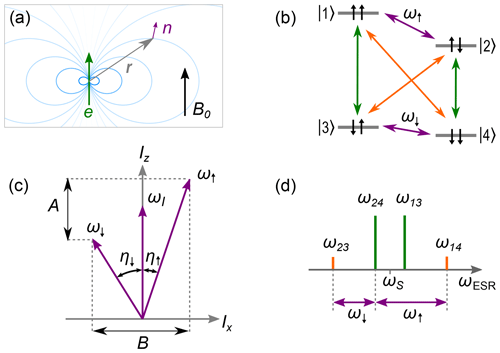Figure 2ESEEM model system for an electron spin of $S=\mathrm{1}/\mathrm{2}$ and nuclear spin of $I=\mathrm{1}/\mathrm{2}$ with ${\mathit{\omega }}_{I},A,B>\mathrm{0}$. (a) Nuclear spin (purple) subject to external field B0 and dipole field (blue) of a nearby electron spin (green) located at relative position r. (b) Energy diagram showing the electron transitions (green), the nuclear transitions (purple), and the (normally forbidden) electro-nuclear transitions (orange). The energy levels $|\mathrm{1}〉,\mathrm{\dots },|\mathrm{4}〉$ are labeled according to the eigenstates of the Zeeman basis. (c) Quantization axes ω and ω due to mixing of the nuclear states, which results in inclination of the quantization axis from z by the angles η and η, respectively. (d) EPR spectrum showing the electron transitions (green) and the electro-nuclear transitions (orange) as well as the relation of these electron spin resonance (ESR) transitions to the nuclear frequencies ω and ω (purple).

Download

Because of the BSzIx term, the nuclear spin is subjected to an effective magnetic field whose direction (and magnitude) depend on the electron-spin state |↑e or |↓e. Its eigenstates therefore depend on the electron-spin state, so that transitions become allowed between all the spin system energy levels |1〉−|4〉, leading to the ESEEM phenomenon. Relevant parameters are the electron-spin-state-dependent angles between the effective magnetic field seen by the nuclear spin and the quantization axis z:

$\begin{array}{}\text{(3)}& \begin{array}{rl}& {\mathit{\eta }}_{↑}=\mathrm{arctan}\frac{B}{A+\mathrm{2}{\mathit{\omega }}_{I}}\\ & {\mathit{\eta }}_{↓}=\mathrm{arctan}\frac{B}{A-\mathrm{2}{\mathit{\omega }}_{I}},\end{array}\end{array}$

and the electron-spin-dependent nuclear-spin frequencies:

$\begin{array}{ll}{\mathit{\omega }}_{↑}& =\left({\mathit{\omega }}_{I}+\frac{A}{\mathrm{2}}\right)\mathrm{cos}{\mathit{\eta }}_{↑}-\frac{B}{\mathrm{2}}\mathrm{sin}{\mathit{\eta }}_{↑}\\ {\mathit{\omega }}_{↓}& =\left({\mathit{\omega }}_{I}-\frac{A}{\mathrm{2}}\right)\mathrm{cos}{\mathit{\eta }}_{↓}-\frac{B}{\mathrm{2}}\mathrm{sin}{\mathit{\eta }}_{↓}.\end{array}$

When η, η are close to equal, only the nuclear-spin-preserving transitions are allowed; this occurs either when B=0 (due to a specific orientation of the dipolar field or a purely isotropic hyperfine coupling), or when B≠0 but ωIA (very weak-coupling limit) or ωIA (very strong coupling limit). On the contrary, when the direction of the effective magnetic field seen by the nuclear spin is electron-spin dependent, all transitions become allowed. This occurs when B≠0 and ${\mathit{\omega }}_{I}\simeq ±A/\mathrm{2}$.

## 2.3 Multi-pulse ESEEM

Because of the level structure shown in Fig. 2, and assuming for simplicity microwave pulses so short that their bandwidth is much larger than ${\mathit{\omega }}_{↑,↓}$, microwave pulses at the electron-spin frequency ωS excite the allowed transitions $|\mathrm{1}〉↔|\mathrm{3}〉$ and $|\mathrm{2}〉↔|\mathrm{4}〉$ but also the normally forbidden $|\mathrm{1}〉↔|\mathrm{4}〉$ and $|\mathrm{2}〉↔|\mathrm{3}〉$, leading to coherence transfer between the levels and to beatings. Note that for simplicity we assume that the microwave pulses are ideal and so short that their bandwidth is much larger than ω12 and ω34.

It is then possible to compute analytically the effect of a two-pulse echo sequence consisting of an instantaneous ideal π∕2 pulse and an instantaneous ideal π pulse (see Fig. 1), disregarding any decoherence. The resulting echo amplitude is given by

$\begin{array}{}\text{(4)}& \begin{array}{rl}{V}_{\mathrm{2}\mathrm{p}}\left(\mathit{\tau }\right)& =\mathrm{1}-\frac{k}{\mathrm{4}}\left[\mathrm{2}-\mathrm{2}\mathrm{cos}\left({\mathit{\omega }}_{↑}\mathit{\tau }\right)-\mathrm{2}\mathrm{cos}\left({\mathit{\omega }}_{↓}\mathit{\tau }\right)\\ & \phantom{\rule{0.25em}{0ex}}+\mathrm{cos}\left(\left({\mathit{\omega }}_{↑}-{\mathit{\omega }}_{↓}\right)\mathit{\tau }\right)+\mathrm{cos}\left(\left({\mathit{\omega }}_{↑}+{\mathit{\omega }}_{↓}\right)\mathit{\tau }\right)\right],\end{array}\end{array}$

with

$\begin{array}{}\text{(5)}& k={\left[\frac{B{\mathit{\omega }}_{I}}{{\mathit{\omega }}_{↑}{\mathit{\omega }}_{↓}}\right]}^{\mathrm{2}}.\end{array}$

The spin-echo amplitude is modulated by a function whose frequency spectrum and amplitude contain information about the nuclear-spin Larmor frequency ωI as well as its hyperfine coupling (A,B) to the electron spin. The modulation contrast $\mathrm{0}\le k\le \mathrm{1}$ is maximal when transitions $|\mathrm{1}〉-|\mathrm{4}〉$ and $|\mathrm{2}〉-|\mathrm{3}〉$ are maximally allowed, corresponding to ${\mathit{\omega }}_{I}\simeq A/\mathrm{2}$.

The above results are exact, as long as the secular approximation is valid and the pulses are ideal. In the weak-coupling limit A, BωI, ${\mathit{\omega }}_{↑}\simeq {\mathit{\omega }}_{↓}\simeq {\mathit{\omega }}_{I}$ so that ${V}_{\mathrm{2}\mathrm{p}}\left(\mathit{\tau }\right)=\mathrm{1}-\frac{k}{\mathrm{4}}\left[\mathrm{3}-\mathrm{4}\mathrm{cos}\left({\mathit{\omega }}_{I}\mathit{\tau }\right)+\mathrm{cos}\left(\mathrm{2}{\mathit{\omega }}_{I}\mathit{\tau }\right)\right]$, with $k=\left(B/{\mathit{\omega }}_{I}{\right)}^{\mathrm{2}}\ll \mathrm{1}$. In this limit, the echo modulation spectrum directly yields the nuclear-spin Larmor frequency and also contains components at twice this frequency. Note however that, in practice, the π pulse bandwidth is always finite, because of the resonator bandwidth or limited pulse power; this sets a limit to the range of detectable modulation frequencies.

The electron spin is often coupled to N nuclear spins, with N>1. Since all nuclear-spin subspaces can be diagonalized separately, the total ESEEM modulation is simply given by the product of each nuclear-spin modulation V2p, l(τ), l being the nuclear-spin index. Taking also into account that the electron spin is also subject to decoherence processes, modeled, for instance, by an exponential decay with time constant T2, the echo envelope is

$\begin{array}{}\text{(6)}& {V}_{\mathrm{2}\mathrm{p}}^{\prime }\left(\mathit{\tau }\right)=\mathrm{exp}\left(-\mathrm{2}\mathit{\tau }/{T}_{\mathrm{2}}\right)\prod _{l=\mathrm{1}}^{N}{V}_{\mathrm{2}\mathrm{p},\phantom{\rule{0.125em}{0ex}}l}\left(\mathit{\tau }\right).\end{array}$

The modulation pattern ${V}_{\mathrm{2}\mathrm{p}}^{\prime }\left(\mathit{\tau }\right)$ yields quantitative information about the nature and coupling of the nuclear spins surrounding the electron spin whose echo is measured and is therefore a useful tool in EPR spectroscopy. When the environmental nuclei have a certain probability p to be of a given isotope with a nuclear spin of $I=\mathrm{1}/\mathrm{2}$, and a probability 1−p to be of an isotope with I=0, the above formulas are straightforwardly modified by writing

$\begin{array}{}\text{(7)}& \begin{array}{rl}{V}_{\mathrm{2}\mathrm{p},\phantom{\rule{0.125em}{0ex}}l}\left(\mathit{\tau }\right)& =\mathrm{1}-\frac{p{k}_{l}}{\mathrm{4}}\left[\mathrm{2}-\mathrm{2}\mathrm{cos}\left({\mathit{\omega }}_{↑,l}\mathit{\tau }\right)-\mathrm{2}\mathrm{cos}\left({\mathit{\omega }}_{↓,l}\mathit{\tau }\right)\\ & \phantom{\rule{0.25em}{0ex}}+\mathrm{cos}\left(\left({\mathit{\omega }}_{↑,l}-{\mathit{\omega }}_{↓,l}\right)\mathit{\tau }\right)+\mathrm{cos}\left(\left({\mathit{\omega }}_{↑,l}+{\mathit{\omega }}_{↓,l}\right)\mathit{\tau }\right)\right].\end{array}\end{array}$

The echo signal ${V}_{\mathrm{2}\mathrm{p}}^{\prime }\left(\mathit{\tau }\right)$ is the sum of terms that have the general form ${p}^{L}{\prod }_{l=\mathrm{1}}^{l=L}{k}_{l}\mathrm{cos}\left({\mathit{\omega }}_{\mathit{\mu },l}\mathit{\tau }\right)$, where l runs over a subset of L nuclei and $\mathit{\mu }=↑,↓$. If p≪1, this expression is well approximated by keeping only the L=1 terms, which then yields

$\begin{array}{}\text{(8)}& \begin{array}{rl}{V}_{\mathrm{2}\mathrm{p}}\left(\mathit{\tau }\right)& \simeq \mathrm{1}-\sum _{l=\mathrm{1}}^{l=N}\frac{p{k}_{l}}{\mathrm{4}}\left[\mathrm{2}-\mathrm{2}\mathrm{cos}\left({\mathit{\omega }}_{↑,l}\mathit{\tau }\right)-\mathrm{2}\mathrm{cos}\left({\mathit{\omega }}_{↓,l}\mathit{\tau }\right)\\ & \phantom{\rule{0.25em}{0ex}}+\mathrm{cos}\left(\left({\mathit{\omega }}_{↑,l}-{\mathit{\omega }}_{↓,l}\right)\mathit{\tau }\right)+\mathrm{cos}\left(\left({\mathit{\omega }}_{↑,l}+{\mathit{\omega }}_{↓,l}\right)\mathit{\tau }\right)\right].\end{array}\end{array}$

One limitation of the previous pulse sequence is that the modulation envelope can only be measured up to a time of order T2 due to electron-spin decoherence, which may be too short for appreciable spectral resolution. This limitation can be overcome by the three-pulse echo sequence shown in Fig. 1b. It consists of a π∕2 pulse applied at t=0 followed, after a time τ chosen such that τ<T2, by a second π∕2 pulse. After a variable delay T, a third π∕2 pulse is applied, leading to the emission of a stimulated echo at time $t=T+\mathrm{2}\mathit{\tau }$. The interest of this sequence is that the first pair of π∕2 pulses generates nuclear-spin coherence that can survive up to the nuclear-spin coherence time T2, n which is in general much longer than T2 (and close to the electron energy spin relaxation time T1). An analytical formula can be derived for the three-pulse echo amplitude in the ideal pulse approximation :

$\begin{array}{}\text{(9)}& \begin{array}{rl}{V}_{\mathrm{3}\mathrm{p}}\left(T\right)& =\mathrm{exp}\left(-T/{T}_{\mathrm{2},\phantom{\rule{0.125em}{0ex}}\mathrm{n}}\right)\mathrm{exp}\left(-\mathrm{2}\mathit{\tau }/{T}_{\mathrm{2}}\right)\\ & \phantom{\rule{0.25em}{0ex}}\mathit{\left\{}\mathrm{1}-\frac{k}{\mathrm{4}}\left[\left[\mathrm{1}-\mathrm{cos}{\mathit{\omega }}_{↓}\mathit{\tau }\right]\left[\mathrm{1}-\mathrm{cos}{\mathit{\omega }}_{↑}\left(T+\mathit{\tau }\right)\right]\\ & \phantom{\rule{0.25em}{0ex}}+\left[\mathrm{1}-\mathrm{cos}{\mathit{\omega }}_{↑}\mathit{\tau }\right]\left[\mathrm{1}-\mathrm{cos}{\mathit{\omega }}_{↓}\left(T+\mathit{\tau }\right)\right]\right]\mathit{\right\}}.\end{array}\end{array}$

Contrary to two-pulse ESEEM, three-pulse echo modulation as a function of T only contains the ${\mathit{\omega }}_{↓},{\mathit{\omega }}_{↑}$ frequency components, and not their sum or difference; that is, in the weak-coupling limit A, BωI, only the nuclear-spin Larmor frequency ωI appears in the spectrum. Another difference is that the modulation pattern and amplitude depend on τ; in particular, its amplitude is zero whenever ${\mathit{\omega }}_{↓,↑}\mathit{\tau }=\mathrm{2}\mathit{\pi }n$ with n integer (“blind spots”).

For weakly coupled nuclei, the modulation amplitude of three-pulse ESEEM can be enhanced by up to 1 order of magnitude by using a more complex pulse sequence known as five-pulse ESEEM and shown in Fig. 1. The analytical formula for the five-pulse echo amplitude V5p is given in the Supplement.

Equation (6), with proper modification to take into account contributions of different pathways, can be applied to the three- and five-pulse ESEEM to treat coupling to multiple nuclear spins. The details are shown in Sect. S3.3 of the Supplement.

## 2.4 Fictitious spin model

The electronic spins that we consider in this work involve an unpaired electron with spin of ${S}_{\mathrm{0}}=\mathrm{1}/\mathrm{2}$ either located around or trapped by an ionic defect, which itself can possess a non-zero nuclear spin of I0. These two spins of the defect are strongly coupled and therefore form a multi-level system, which can nevertheless be mapped to an effective, fictitious, spin-1∕2 model as explained below , to which the model of Sect. 2.3 can be applied.

The system spin Hamiltonian writes

$\begin{array}{}\text{(10)}& {\mathbf{H}}_{\mathrm{ion}}={\mathit{\beta }}_{\mathrm{e}}{\mathbit{B}}_{\mathbf{0}}\cdot {\mathbf{g}}_{\mathrm{e}}\cdot {\mathbf{S}}_{\mathrm{0}}+{\mathbf{S}}_{\mathrm{0}}\cdot {\mathbf{A}}_{\mathrm{0}}\cdot {\mathbf{I}}_{\mathrm{0}}.\end{array}$

Here, βe is the electron Bohr magneton, ge is the (possibly anisotropic) gyromagnetic tensor, and A0 the hyperfine tensor. The nuclear Zeeman interaction of the defect system, being small compared to the hyperfine interaction in the range of magnetic fields explored here, is neglected from the Hamiltonian.

This multi-level electron-spin system is coupled to other nuclear spins in the lattice, giving rise to ESEEM. Consider a nuclear spin at a lattice site j, defined by its location rj with respect to the electron spin. The nuclear Zeeman Hamiltonian is ${\mathbf{H}}_{j}={\mathit{\omega }}_{I}{\mathbf{I}}_{j,z}$, with ωI=gnβnB0, gn being the nuclear g factor and βn the nuclear magneton. Its hyperfine coupling to the electron-spin system is described by the Hamiltonian:

$\begin{array}{}\text{(11)}& {\mathbf{H}}_{j,\phantom{\rule{0.125em}{0ex}}\mathrm{hf}}={\mathbf{S}}_{\mathrm{0}}\cdot {\mathbf{A}}_{j}\cdot {\mathbf{I}}_{j},\end{array}$

with

$\begin{array}{}\text{(12)}& {\mathbf{A}}_{j}={\mathbf{A}}_{j,\phantom{\rule{0.125em}{0ex}}\mathrm{cf}}+{\mathbf{A}}_{j,\phantom{\rule{0.125em}{0ex}}\mathrm{dd}}.\end{array}$

This hyperfine tensor consists of a Fermi contact term, ${\mathbf{A}}_{j,\phantom{\rule{0.125em}{0ex}}\mathrm{cf}}=\frac{\mathrm{2}}{\mathrm{3}}\phantom{\rule{0.125em}{0ex}}{\mathit{\mu }}_{\mathrm{0}}\phantom{\rule{0.125em}{0ex}}{\mathit{\beta }}_{\mathrm{e}}\phantom{\rule{0.125em}{0ex}}{g}_{\mathrm{n}}\phantom{\rule{0.125em}{0ex}}{\mathit{\beta }}_{\mathrm{n}}\phantom{\rule{0.125em}{0ex}}{\mathbf{g}}_{\mathrm{e}}{\left|\mathit{\psi }\left({\mathbit{r}}_{j}\right)\right|}^{\mathrm{2}}$, and a dipole–dipole term, ${\mathbf{A}}_{j,\phantom{\rule{0.125em}{0ex}}\mathrm{dd}}=\frac{\mathrm{3}{\mathit{\mu }}_{\mathrm{0}}}{\mathrm{4}\mathit{\pi }{\left|{\mathbit{r}}_{j}\right|}^{\mathrm{5}}}{\mathit{\beta }}_{\mathrm{e}}\phantom{\rule{0.125em}{0ex}}{\mathit{\beta }}_{\mathrm{n}}\phantom{\rule{0.125em}{0ex}}{g}_{\mathrm{n}}\left[{\mathbit{r}}_{j}^{\mathrm{2}}{\mathbf{g}}_{\mathrm{e}}-\mathrm{3}\left({\mathbf{g}}_{\mathrm{e}}\cdot {\mathbit{r}}_{j}\right){\mathbit{r}}_{j}\right]$, ψ(rj), being the electron wave function at the nuclear-spin location.

The Hamiltonian Hion (Eq. 10) can be diagonalized, yielding 4I0+2 energy levels. It is in general possible to isolate two levels |α and |β that are coupled by an electron spin resonance (ESR)-allowed transition and are resonant or quasi-resonant with the microwave cavity, with a transition frequency ωS. If these two levels are sufficiently separated in energy from other levels of Hion, they define a fictitious $S=\mathrm{1}/\mathrm{2}$ system. Writing the total Hamiltonian ${\mathbf{H}}_{\mathrm{ion}}+{\mathbf{H}}_{j}+{\mathbf{H}}_{j,\phantom{\rule{0.125em}{0ex}}\mathrm{hf}}$ restricted to this two-dimensional subspace yields

$\begin{array}{}\text{(13)}& \begin{array}{rl}{\mathbf{H}}_{\mathrm{0}}& ={\mathit{\omega }}_{S}{\mathbf{S}}_{z}+\left({\mathit{\omega }}_{I}+\frac{{m}_{S}^{\mathit{\alpha }}+{m}_{S}^{\mathit{\beta }}}{\mathrm{2}}{\mathbf{A}}_{j,zz}\right){\mathbf{I}}_{j,z}\\ & +\frac{{m}_{S}^{\mathit{\alpha }}+{m}_{S}^{\mathit{\beta }}}{\mathrm{2}}{\mathbf{A}}_{j,zx}{\mathbf{I}}_{j,x}\\ & +\left({m}_{S}^{\mathit{\alpha }}-{m}_{S}{}^{\mathit{\beta }}\right)\left({\mathbf{A}}_{j,zz}{\mathbf{S}}_{z}{\mathbf{I}}_{j,z}+{\mathbf{A}}_{j,zx}{\mathbf{S}}_{z}{\mathbf{I}}_{j,x}\right)\phantom{\rule{0.125em}{0ex}},\end{array}\end{array}$

where ${m}_{S}^{\mathit{\alpha },\phantom{\rule{0.125em}{0ex}}\mathit{\beta }}=〈\mathit{\alpha },\mathit{\beta }|{\mathbf{S}}_{\mathrm{0},z}|\mathit{\alpha },\mathit{\beta }〉$.

Equation (13) maps the more complex system to the simple model of Sect. 2.2. Compared to Eq. (2), two differences appear. First, the hyperfine interaction parameters A, B are rescaled by the effective longitudinal magnetization difference $\left({m}_{S}^{\mathit{\alpha }}-{m}_{S}^{\mathit{\beta }}\right)$ which depends on the two levels considered. Second, when the average longitudinal magnetization of the two levels $\left({m}_{S}^{\mathit{\alpha }}+{m}_{S}^{\mathit{\beta }}\right)$ is non-zero, the nuclear spin sees an extra Zeeman contribution which may be tilted with respect to the z axis. Once taken into account, these corrections, the analysis, and formulas of Sect. 2.3 remain valid.

3 Spin systems

## 3.1 Erbium-doped CaWO4

The first system investigated consists of erbium Er3+ ions doped into a CaWO4 matrix, substituting Ca2+. The crystal has a tetragonal body-centered structure (see Fig. 3) with lattice constants $a=b=\mathrm{0.524}$ nm and c=1.137 nm. Rare-earth ions with an odd number of electrons such as Er3+ have a ground state consisting of two levels that are degenerate in zero magnetic field and separated from other levels by an energy scale equivalent to several tens of Kelvin due to the crystalline electric field and the spin–orbit interaction. This pair of electronic levels is known as a Kramers doublet and forms an effective ${S}_{\mathrm{0}}=\mathrm{1}/\mathrm{2}$ electron-spin system, with a spin Hamiltonian HEr whose form is given by Eq. (10).

Due to the S4 site symmetry in which rare-earth ions are found in CaWO4, the g tensor is diagonal in the crystallographic frame with ${g}_{xx}={g}_{yy}=\mathrm{8.38}$ and gzz=1.247 (x, y, z corresponding to a, b, c). Of all erbium atoms, 77 % are from an isotope that has nuclear spin of I0=0 and therefore no contribution from the hyperfine term in Eq. (10). Their energy levels are shown in Fig. 3 for B0 applied in the (a,b) plane.

The remaining 23 % are from the 167Er isotope with ${I}_{\mathrm{0}}=\mathrm{7}/\mathrm{2}$. Its hyperfine coupling tensor to the Er3+ electron spin is diagonal, with coefficients ${A}_{xx}={A}_{yy}=\mathrm{873}$ MHz and Azz=130 MHz. The 16 eigenfrequencies of the 167Er spin Hamiltonian are also shown in Fig. 3, again for B0 applied in the (a,b) plane. In the high-magnetic-field limit of ${B}_{\mathrm{0}}\gg {A}_{\mathrm{Er}}/\left({g}_{\mathrm{Er}}{\mathit{\beta }}_{\mathrm{e}}\right)$, the eigenstates are simply described by $|±,{m}_{I}〉$, ± describing the electron-spin quantum number ${m}_{S}=±\mathrm{1}/\mathrm{2}$ and mI the nuclear-spin quantum number. For B0<100 mT, as is the case in the measurements described here, this limit is only approximate, but we will nevertheless use the high-field state vectors as labels for the lower-field eigenstates. The strongest EPR-allowed transitions are the mI-preserving transitions. In the following, we will apply the fictitious spin model with $|\mathit{\alpha },\mathit{\beta }〉$ = $|±,{m}_{I}〉$.

The CaWO4 matrix also contains nuclear spins. Indeed, the 183W isotope has a spin of $I=\mathrm{1}/\mathrm{2}$ with nuclear g factor gn=0.235 (corresponding to a gyromagnetic ratio of 1.8 MHz T−1) and is present in a p=0.13 abundance, whereas the other tungsten isotopes are nuclear-spin free. The interaction of the 183W atoms with the erbium ions gives rise to the ESEEM studied below. Because the 4f electron wave function is mainly located on the Er3+ ion, the contact hyperfine with the nuclear spins of the lattice is expected to be negligibly small. We therefore model the hyperfine interaction with 183W by the dipole–dipole term in Eq. (12).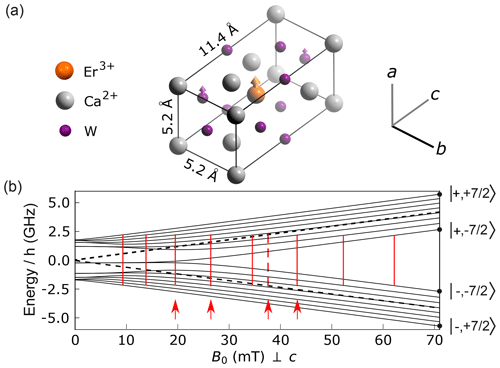Figure 3Structure and energy diagram of erbium ions in CaWO4. (a) Crystal structure with oxygen atoms hidden for clarity. Erbium atoms are in substitution of the calcium. The crystal has a rotational symmetry around the c axis. A fraction p=0.13 of the W atoms are of the 183W isotope, with a nuclear spin of 1∕2. (b) Energy level diagram of the I=0 erbium isotopes (dashed black line) and of the 167Er isotope (solid black lines) with $I=\mathrm{7}/\mathrm{2}$ for B0 applied perpendicular to the c axis. Vertical red lines indicate the value of B0 for which an allowed EPR transition becomes resonant with the 4.372 GHz frequency of our detection resonator (see main text, Sect. 4). Four red arrows indicate the values of B0 at which ESEEM data were measured.

Download

## 3.2 Bismuth donors in silicon

The other system considered is the bismuth donor in silicon. Bismuth, as an element of the fifth column, substitutes in the silicon lattice by making four covalent bonds with neighboring atoms, leaving one unpaired electron that can be weakly trapped by the hydrogenic potential generated by the Bi+ ion, whose spin gives rise to the resonance signal (see Fig. 4a). The donor wave function ψ(r) has a complex structure that extends over ≈1.5 nm in the silicon lattice (see the Supplement). As for Er:CaWO4, the donor spin Hamiltonian HBi is given by Eq. (10). However, in this case, the g tensor ge1 is isotropic with ge=2, and the hyperfine tensor ABi1 with the nuclear spin of ${I}_{\mathrm{0}}=\mathrm{9}/\mathrm{2}$ of the bismuth atom is also isotropic, with ${A}_{\mathrm{Bi}}/\mathrm{2}\mathit{\pi }=\mathrm{1.4754}$ GHz.

The eigenstates of HBi have simple properties because of its isotropic character. Denoting mS (mI) the eigenvalue of Sz,0 (Iz,0), we note that $m={m}_{I}+{m}_{S}$ is a good quantum number since HBi commutes with ${\mathbf{S}}_{z,\mathrm{0}}+{\mathbf{I}}_{z,\mathrm{0}}$ , z being the direction of B0. States with equal m are hybridized by HBi. States $|m=\mathrm{5}〉$ and $|m=-\mathrm{5}〉$, corresponding to $|{m}_{S}=+\mathrm{1}/\mathrm{2},{m}_{I}=\mathrm{9}/\mathrm{2}〉$ and $|{m}_{S}=-\mathrm{1}/\mathrm{2},{m}_{I}=-\mathrm{9}/\mathrm{2}〉$, are non-degenerate and are thus also eigenstates of HBi. States with $|m|\le \mathrm{4}$ belong to nine two-dimensional subspaces spanned by $|{m}_{S}=+\mathrm{1}/\mathrm{2},{m}_{I}=m-\mathrm{1}/\mathrm{2}〉,|{m}_{S}=-\mathrm{1}/\mathrm{2},{m}_{I}=m+\mathrm{1}/\mathrm{2}〉$ within which the two eigenstates of HBi are given by $|±,m〉={a}_{m}^{±}|±\frac{\mathrm{1}}{\mathrm{2}},m\mp \frac{\mathrm{1}}{\mathrm{2}}〉+{b}_{m}^{±}|\mp \frac{\mathrm{1}}{\mathrm{2}},m±\frac{\mathrm{1}}{\mathrm{2}}〉$, with values of ${a}_{m}^{±},{b}_{m}^{±}$ that can be determined analytically .

Contrary to the erbium case, the measurements of bismuth donor spins are performed in the low-field limit |geβeB0|≪|ABi|, in which the eigenstates are fully hybridized. In this limit, a useful approximate expression for the eigenenergy of level $|±,m〉$ is

$\begin{array}{}\text{(14)}& {E}_{m}^{±}\approx -\frac{{A}_{\mathrm{Bi}}}{\mathrm{2}}±\frac{\mathrm{5}{A}_{\mathrm{Bi}}}{\mathrm{2}}±\frac{m{g}_{\mathrm{e}}{\mathit{\beta }}_{\mathrm{e}}|{\mathbit{B}}_{\mathrm{0}}|}{\mathrm{10}}.\end{array}$

The magnetic-field dependence of the $|±,m〉$ energy levels is shown in Fig. 4b for B0<1 mT. Note in particular that the separation between neighboring hyperfine levels is given by ${E}_{m}^{±}-{E}_{m-\mathrm{1}}^{±}\approx ±\frac{{g}_{\mathrm{e}}{\mathit{\beta }}_{\mathrm{e}}{B}_{\mathrm{0}}}{\mathrm{10}}=±\mathrm{2}\mathit{\pi }×\mathrm{2.8}\phantom{\rule{0.125em}{0ex}}{B}_{\mathrm{0}}$ GHz.

Because of the hybridization, all transitions that satisfy $|\mathrm{\Delta }m|=\mathrm{1}$ are to some extent EPR allowed at low field; i.e., they have a non-zero matrix element of operator S0,x. In this work, we particularly focus on the 18 $|\mathrm{\Delta }m|=\mathrm{1}$ transitions that are in the ≃7 GHz frequency range at low magnetic fields $|+,m〉↔|-,m-\mathrm{1}〉$ and $|-,m〉↔|+,m-\mathrm{1}〉$, as shown in Fig. 4c. The $|-,m〉↔|+,m+\mathrm{1}〉$ and $|-,m+\mathrm{1}〉↔|+,m-\mathrm{1}〉$ transitions are degenerate in frequency for $-\mathrm{4}\le m<\mathrm{4}$ as seen from Eq. (14), which results in only 10 different transition frequencies (see Figs. 4b–c and 8a).

The most abundant isotope of silicon is 28Si, which is nuclear-spin free. The lattice also contains a small percentage p of 29Si atoms that have a nuclear spin of $I=\mathrm{1}/\mathrm{2}$ and give rise to the ESEEM. The g factor of 29Si is ${g}_{\mathrm{n}}=-\mathrm{1.11}$, yielding a gyromagnetic ratio of 8.46 MHz T−1.

The donor–29Si hyperfine interaction is given by Eq. (12). Due to the spatial extent of the electron wave function, the Fermi contact term is not negligible and needs to be taken into account together with the dipole–dipole coupling ; more details can be found in the Supplement.

The restriction of the total system Hamiltonian to each of the 18 ESR-allowed transitions of the bismuth donor manifold can be mapped onto the fictitious spin-1∕2 model of Sect. 2.4. Note however that the hyperfine term $|{A}_{j}|$ can take values up to ∼1 MHz for proximal nuclear spins, which is comparable to or larger than the frequency difference between hyperfine states of the bismuth donor manifold at low field as explained above. The validity of the fictitious spin-1∕2 model in this context will be discussed in Sect. 5.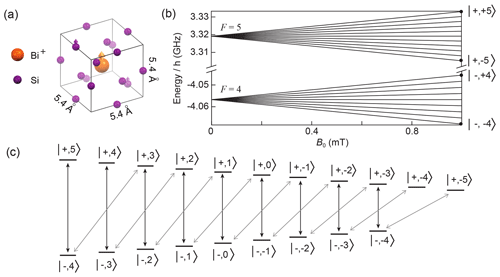Figure 4Structure and energy diagram of bismuth donors in silicon. (a) Silicon crystal structure, showing a substitutional bismuth atom coupled to nearby 29Si nuclear spins. The donor electron is trapped around the Bi+ ion and its wave function covers many lattice sites. (b) Energy levels of the bismuth donor for B0<1 mT. (c) Schematic representation of the allowed transitions (black and grey arrows) between the bismuth donor energy levels in the low field limit.

Download

4 Experimental setup and samples

The EPR spectrometer has been described in detail in and and is shown schematically in Fig. 5a. It is built around a superconducting micro-resonator of frequency ωr consisting of a planar interdigitated capacitor shunted by an inductor, directly patterned on the crystal. We detect the spins that are located in the immediate vicinity of the resonator inductance. Note that the microwave B1 field generated by the inductance is spatially inhomogeneous. If the spin location is broadly distributed, this can make the application of control pulses with a well-defined Rabi angle problematic . As explained below, the resonator is more strongly coupled to the measurement line than in to increase the measurement bandwidth as requested for ESEEM spectroscopy.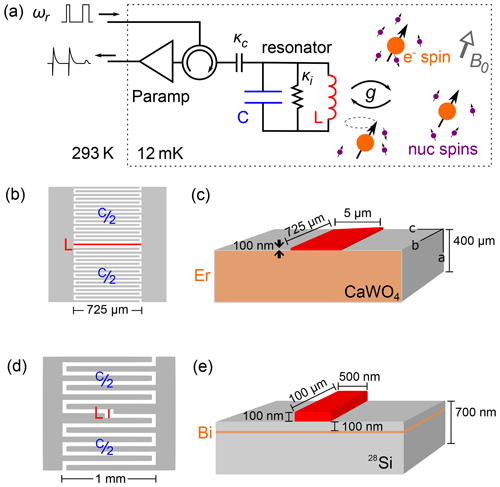Figure 5Experimental setup and samples. (a) Schematic of the low-temperature EPR spectrometer. The LC resonator is inductively coupled to electron spins, which are coupled to a nuclear-spin bath that causes the ESEEM. The spins are probed by sequences of microwave pulses at the resonator frequency ${\mathit{\omega }}_{\mathrm{r}}=\mathrm{1}/\sqrt{\text{LC}}$. Reflected pulses as well as the echo signal are routed to a parametric amplifier, further amplified at 4 K, and finally demodulated and digitized at room temperature. (b, c) Design of the LC resonator used for the detection of erbium ion spins, with a 725 µm long, 5 µm wide inductor. It is patterned out of a 100 nm thick niobium film deposited on top of a CaWO4 substrate bulk doped with Er3+ ions. (d, e) Design of the LC resonator used for the detection of bismuth donor spins, with a 100 µm long, 0.5 µm wide inductor. It is patterned out of a 100 nm thick aluminum film deposited on top of a silicon substrate isotopically enriched in 28Si, in which bismuth ions were implanted at a 50–100 nm depth.

Download

The sample is mounted in a copper sample holder thermally anchored at the mixing chamber of a dilution refrigerator. A DC magnetic field B0 is applied parallel to the sample surface and along the resonator inductance. The resonator is coupled capacitively to an antenna, which is itself connected to a microwave measurement setup in reflection. To minimize heat load, the coaxial cables between 4 K and 10 mK are in superconducting niobium–titanium (NbTi). To suppress thermal noise, the input line is heavily attenuated at low temperatures. Microwave pulses for driving the spins are sent to the resonator input, and their reflection or transmission, together with the echo signal emitted by the spins, is fed into a superconducting JPA, either of the flux-pumped type or of the Josephson traveling-wave parametric amplifier (JTWPA) type . Further microwave amplification takes place at 4 K with a high-electron-mobility transistor (HEMT) from Low Noise Factory, and then at room temperature, before homodyne demodulation which yields the two signal quadratures [I(t),Q(t)]. The echo-containing quadrature signal is integrated to yield the echo amplitude Ae. Such a setup was shown to reach sensitivities of the order of 102103 spin/$\sqrt{\mathrm{Hz}}$ .

Because of the small resonator mode volume and high quality factor, little microwave power is needed to drive the spins. The exact amount depends on the resonator geometry, as conveniently expressed by the power-to-field conversion factor $\mathit{\alpha }={B}_{\mathrm{1}}/\sqrt{{P}_{\mathrm{in}}}$. In the experiments reported here, the maximum microwave power used to drive the spins is on the order of 10 nW. At this power, the superconducting pre-amplifiers saturate; however, they recover rapidly enough (within a few microseconds) to amplify the much weaker subsequent spin echoes. Flux-pumped JPAs are moreover switched off during the control pulses by pulsing the pump tone, whereas the JTWPA was kept on all the time. All microwave powers reaching the 4 K HEMT are low enough that neither saturation nor damage are to be expected at this stage.

The erbium-doped sample (from Scientific Materials) was prepared by mixing erbium oxide with calcium and tungsten oxides before crystal growth, yielding a uniform Er concentration of 6×1017 cm−3 (50 ppm) throughout the sample. For resonator fabrication, the bulk crystal was cut and polished to a thin rectangular sample with dimensions $\mathrm{0.4},\mathrm{mm}×\mathrm{3}\phantom{\rule{0.125em}{0ex}}\mathrm{mm}×\mathrm{6},\mathrm{mm}$ parallel to $a×b×c$ axes. The resonator was patterned out of a 100 nm thick (sputtered) niobium (Nb) layer, using a design similar to that shown in . More specifically, 15 interdigitated fingers on either side of a 720 µm×5  µm inductive wire form an LC resonator, corresponding to a detection volume of VEr∼20 pL. In the absence of magnetic field, the resonance frequency is ${\mathit{\omega }}_{\mathrm{r}}/\mathrm{2}\mathit{\pi }=\mathrm{4.323}$ GHz. Its total quality factor of 8×103 is set both by the internal losses, characterized by the energy loss rate ${\mathit{\kappa }}_{\mathrm{i}}=\mathrm{5}×{\mathrm{10}}^{\mathrm{5}}$ s−1, and by its coupling to the measurement line ${\mathit{\kappa }}_{\mathrm{C}}=\mathrm{3}×{\mathrm{10}}^{\mathrm{6}}$ s−1. For this geometry, the power-to-field factor is α=1.7 T W${}^{-\mathrm{1}/\mathrm{2}}$.

The bismuth donors have been implanted at ≈100 nm depth with a peak concentration of 8×1016 cm−3 in a silicon sample. They lie in a 700 nm thick silicon epilayer enriched in the nuclear-spin-free 28Si isotope (nominal concentration of 99.95 %), grown on top of a natural-abundance silicon sample. The resonator is patterned out of a 50 nm thick aluminum film. It has the same geometry as reported in , with a 100 µm long, 500 nm wide inductor, and a detection volume of 0.2 pL. Its frequency ${\mathit{\omega }}_{\mathrm{r}}/\mathrm{2}\mathit{\pi }=\mathrm{7.370}$ GHz is only slightly below the zero-field splitting of unperturbed Bi:Si donors at $\mathrm{5}{A}_{\mathrm{Bi}}/\left(\mathrm{2}\mathit{\pi }\right)=\mathrm{7.37585}$ GHz . The resonator internal loss is given by ${\mathit{\kappa }}_{\mathrm{i}}=\mathrm{3}×{\mathrm{10}}^{\mathrm{5}}$ s−1. The coupling to the measurement line can be tuned at will by modifying the length of a microwave antenna that capacitively couples the measurement waveguide to the on-chip resonator via the copper sample holder . For the experiments reported below, we used two settings: one for which the resonator was overcoupled (κC1=107 s−1), corresponding to a loaded quality factor of ${Q}_{\mathrm{1}}=\mathrm{4}×{\mathrm{10}}^{\mathrm{3}}$, and one for which the coupling was closer to critical (κC2=106 s−1), corresponding to a loaded quality factor of ${Q}_{\mathrm{2}}=\mathrm{3.4}×{\mathrm{10}}^{\mathrm{4}}$. In the low-Q case, square microwave pulses were used, of duration ≃100 ns similar to the cavity field damping time. In the high-Q case, shaped pulses were used so that the intra-cavity field was a square pulse of 1 µs without any ringing. In some experiments, we additionally used a train of π pulses (Carr–Purcell–Meiboom–Gill sequence; CPMG), which generated extra echoes for significant gain in signal-to-noise ratio. More details on the pulse sequences used, the phase cycling scheme, and the repetition time, will be given in the following sections, together with experimental results. For this geometry, the power-to-field factor is α=9 T W${}^{-\mathrm{1}/\mathrm{2}}$ for the low-Q case, and α=21 T W${}^{-\mathrm{1}/\mathrm{2}}$ for the high-Q case.

5 Results

## 5.1 Erbium-doped CaWO4

### 5.1.1 Spectroscopy

Figure 6 shows a spectrum comprising a series of microwave transmission measurements recorded on a vector network analyzer, measured at 100 mK, as a function of the magnetic field B0 applied along the b crystal axis . Note that compared to Fig. 5a, the resonator is coupled to the measurement line in a hanger geometry , so that its resonance appears as a dip in the amplitude transmission coefficient $|{S}_{\mathrm{21}}|$ (see Fig. 6). The nine red lines indicate the values of B0 at which the calculated Er3+ ion transitions are equal to ωr (see Fig. 3b). Avoided level crossings are observed, which indicate a strong coupling of the resonator to the erbium transitions. Several additional anti-crossings and discontinuities are visible above 40 mT. These are attributed to ytterbium impurities 171Yb and 173Yb and magnetic flux vortices penetrating the resonator.

Noticeable in the spectrum at 37 mT is the large anti-crossing attributed to the highly concentrated I=0 erbium isotopes. Here, the high-cooperativity regime (C>30) is reached between the electronic spins and the resonator . Typical linewidth of $\mathrm{\Gamma }/\mathrm{2}\mathit{\pi }\sim \mathrm{20}$ MHz is observed. The coupling strength is also observed to be different for the eight 167Er transitions, which are labeled according to their corresponding nuclear-spin projections mI. This is explained by the partial polarization of the ground-state hyperfine levels of 167Er3+ at millikelvin temperatures (see Fig. 3b).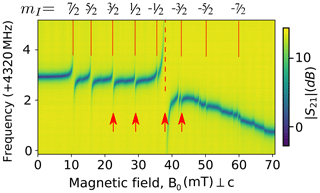Figure 6Spectroscopy of ${\mathrm{Er}}^{\mathrm{3}+}:{\mathrm{CaWO}}_{\mathrm{4}}$. Transmission coefficient $|{S}_{\mathrm{21}}|\left(\mathit{\omega }\right)$ at 100 mK as a function of the magnetic field B0 applied along to the a crystalline axis, around 4.323 GHz. Vertical red lines indicate the expected erbium transitions either for the I=0 isotopes (dashed) or the $I=\mathrm{7}/\mathrm{2}$ isotope (solid). Red arrows indicate the field at which the ESEEM data are measured.

Download

### 5.1.2 Two-pulse ESEEM

Four values of B0 were selected for investigating ESEEM, indicated by the arrows in Fig. 6; the first, second, and fourth corresponding to electronic-spin transitions of 167Er, and the third one to the I=0 isotopes. The two-pulse echo sequence of Fig. 1a was implemented with square pulses of 1µs duration applied at the resonator input, with double amplitude for the second pulse. Note that due to the B1 spatial inhomogeneity combined with the homogeneous spin distribution throughout the crystal, the spread of Rabi frequency is too large to observe a well-defined nutation signal. The Rabi angle is therefore not well defined, and the echo is the average of different rotation angles.

The control pulses driving the spins are filtered by the resonator bandwidth $\mathit{\kappa }/\mathrm{2}\mathit{\pi }\simeq \mathrm{600}$ kHz, corresponding to a field decay time $\mathrm{2}{\mathit{\kappa }}^{-\mathrm{1}}=\mathrm{3.3}$µs. The repetition time between echo sequences was 1 s, close to the spin relaxation time T1∼1–2 s measured by saturation recovery on the transitions studied. The echo signal was averaged 10 times with phase cycling of the π pulse to improve signal-to-noise ratio and to remove signal offsets.

Figure 7 shows the two-pulse echo integrated amplitude Ae as a function of τ for each of the four Er transitions investigated . A clear envelope modulation signal is observed, together with an overall damping. Here, we are interested only in the modulation pattern; a detailed study of the coherence time T2 will be provided elsewhere. Qualitatively, we observe that the modulation frequency increases with B0 and the modulation amplitude overall decreases with B0, as expected from the discussion in Sect. 2. A Fourier transform of the I=0 data (see Fig. 7b) shows the ESEEM spectrum. Well-resolved peaks are observed in the 5–100 kHz range, distributed around the 183W bare Larmor frequency ωW.

A very rough estimate of the number of erbium ions contributing to the signal is [Er]VErκ∕Γ, which is 2.5×108 for the I=0 data, and 107 for each 167Er transition.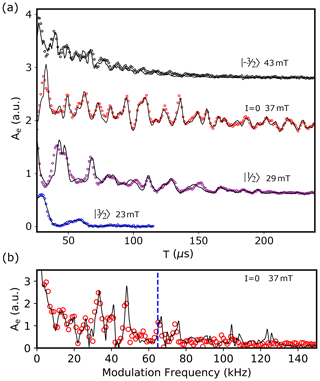Figure 7Two-pulse ESEEM on Er:CaWO4. (a) Integrated echo area Ae as a function of the inter-pulse delay τ for four values of B0 corresponding to different transitions. Open circles are measurements, and solid lines are the results of the ESEEM calculations as explained in Sect. S5.1. (b) Measured (open red circles) and computed (solid line) fast Fourier transform of the I=0 data. The dashed blue line shows the Larmor frequency of 183W nuclei in free space.

Download

### 5.1.3 Comparison with the model

We compute the echo envelope ${V}_{\mathrm{2}\mathrm{p}}^{\prime }\left(\mathit{\tau }\right)$ described in Sect. 2.3, with the nearest 1000 coupled tungsten nuclei (N=1000) and a natural 183W abundance of 14.4 % (p=0.144). The hyperfine interaction is taken to be purely dipolar, as already explained . The fitting proceeds by assigning an initial “guess” to six free parameters, then minimizing using the limited-memory Broyden–Fletcher–Goldfarb–Shanno algorithm for bound-constrained optimization (L-BFGS-B) . Three of these parameters $\left(|{B}_{\mathrm{0}}|,\mathit{\varphi },\mathit{\theta }\right)$ describe the applied magnetic field:

${\mathbit{B}}_{\mathrm{0}}=|{\mathbit{B}}_{\mathrm{0}}|\left[\mathrm{sin}\mathit{\theta }\mathrm{cos}\mathit{\varphi }\phantom{\rule{0.125em}{0ex}}\mathbit{x}+\mathrm{sin}\mathit{\theta }\mathrm{sin}\mathit{\varphi }\phantom{\rule{0.125em}{0ex}}\mathbit{y}+\mathrm{cos}\mathit{\theta }\phantom{\rule{0.125em}{0ex}}\mathbit{z}\right].$

Here, θ is the angle of the field relative to the crystal c axis (z) and ϕ is the angle relative to the a axis (x) in the ab plane (xy plane). The other three parameters (C, T2, n) account for the echo envelope decay:

$\mathrm{1}{A}_{\mathrm{e}}\left(\mathit{\tau }\right)={V}_{\mathrm{2}\mathrm{p}}\left(\mathit{\tau }\right)\cdot C\mathrm{exp}{\left(-\frac{\mathrm{2}\mathit{\tau }}{{T}_{\mathrm{2}}}\right)}^{n},$

where C represents the signal magnitude, T2 represents the coherence time, and $n\in \left[\mathrm{1},\mathrm{2}\right]$ accounts for non-exponential decay. To determine the global minimum of the fit, the minimization is repeated 200 times with randomly seeded initial values for the six parameters, bounded within the known uncertainty of the applied magnetic field B0, signal strength C, and coherence time T2. This approach reveals single local minima for each fitted parameter within the bounded range, with the variance of the 200 outcomes determining the uncertainty for each parameter. In particular, it yields precise values for the angles $\mathit{\theta }=\mathrm{91.47}±\mathrm{0.01}$ and $\mathit{\varphi }=\mathrm{90.50}±\mathrm{0.01}$. The result of this fitting is presented in Fig. 7a, overlaid on the data for the I=0 transition at 37 mT. Only the decay parameters (C, T2, n) and magnetic-field magnitude $|{B}_{\mathrm{0}}|$ are left free when fitting the other three transitions in Fig. 7a. This was done for consistency between datasets, and because the I=0 data yield the most accurate values for ϕ and θ due to the low decoherence rate. The fits yield coherence times T2 varying between 40 and 400 µs, depending on the transition considered. Good agreement was also reached between the fitted and expected (pre-calibrated) field magnitudes.

Note that good fits to the data are also achieved by including only the nearest 100 tungsten nuclei, although noticeable deviations between the data and fit are observed with any amount less. The dimensionless “anisotropic hyperfine interaction parameter” ρ described in the seminal publication on ESEEM is not required here. This parameter was introduced with the earliest attempts of ESEEM fitting, likely to compensate for the low number of simulated nuclear spins (typically 10 nearest nuclei or less) and was interpreted as an account for a potential distortion of the local environment caused by dopant insertion. Finally, a consideration of the spectral components presented in Fig. 7b helps to more clearly identify the difference between the fit and the data. In particular, the high-frequency components of the fitted model are not present experimentally due to the filtering effect of the superconducting resonance (260 kHz half width at half maximum; HWHM). This high-Q resonator greatly reduces the bandwidth of the radio frequency (RF) field absorbed by the coupled Er−183W system and further limits the bandwidth of the detected echo signal.

## 5.2 Bismuth donor sample

### 5.2.1 Spectroscopy

Given the resonator frequency ωr, four bismuth donor resonances should be observed when varying B0 between 0 and 1 mT, as seen in Fig. 8a. Figure 8b shows an echo-detected field sweep, measured at 12 mK: the integrated area Ae of echoes obtained with a sequence shown in Fig. 1a with τ=50µs pulse separation is plotted as a function of B0  . Instead of showing well-separated peaks as in the erbium case, echoes are observed for all fields below 1 mT, with a maximum close to 0.1 mT, and extends in particular down to B0=0 mT . This is the sign that each of the expected peaks is broadened and overlaps with neighboring transitions. Close to zero field, the echo amplitude goes down by a factor of 2 on a scale of ∼0.1 mT, before showing a sharp increase at exactly zero field. These zero-field features are not currently understood, but they are reproducible as confirmed by the measurements at B0<0, which are approximately symmetric to the B0>0 data as they should be.

Line broadening was reported previously for bismuth donors in silicon in related experiments and was attributed to the mechanical strain exerted by the aluminum resonator onto the silicon substrate due to differential thermal contractions between the metal and the substrate. At low strain, ABi depends linearly on the hydrostatic component of the strain tensor ${\mathit{ϵ}}_{\mathrm{hs}}=\left({\mathit{ϵ}}_{xx}+{\mathit{ϵ}}_{yy}+{\mathit{ϵ}}_{zz}\right)/\mathrm{3}$ with a coefficient $\mathrm{d}{A}_{\mathrm{Bi}}/\mathrm{d}{\mathit{ϵ}}_{\mathrm{hs}}/\left(\mathrm{2}\mathit{\pi }\right)=\mathrm{28}$ GHz . Quantitative understanding of the line shape was achieved in a given sample geometry based on this mechanism , using a finite-element modeling to estimate the strain profile induced upon sample cooldown. A similar modeling was performed for the Bi sample reported here (see Fig. 8d). Based on the typical strain distribution $|{\mathit{ϵ}}_{\mathrm{hyd}}|\sim \mathrm{3}×{\mathrm{10}}^{-\mathrm{4}}$ and on the hyperfine to strain coefficient $\mathrm{d}{A}_{\mathrm{Bi}}/\mathrm{d}{\mathit{ϵ}}_{\mathrm{hs}}/\left(\mathrm{2}\mathit{\pi }\right)=\mathrm{28}$ GHz, we expect the zero-field splitting 5ABi∕(2π) to have a spread of ∼50 MHz, which would indeed result in complete peak overlap in the B0<1 mT region, as observed in Fig. 8b.

This broadening has two consequences worth highlighting. First, the bismuth donor echo signals can be measured down to B0=0 mT, which otherwise is generally impossible in X-band spectroscopy. Here, this is enabled by the large hyperfine coupling of the Bi:Si donor, combined with strain-induced broadening. This makes it possible to detect ESEEM caused by very weakly coupled nuclear spins, which requires low magnetic fields as explained in Sect. 2. Second, at a given magnetic field, the spin-echo signal contains contributions from several overlapping EPR transitions. This last point is best understood from Fig. 8c, which shows how several classes of bismuth donors, each with different hyperfine coupling ABi, may have transitions resonant with ωr. We will assume in the following that the inhomogeneous distribution of ABi is so broad that each of the 10 ABi values for which one bismuth donor transition is resonant with ωr at fixed B0 is equally probable, which is likely to be valid for B0<1 mT.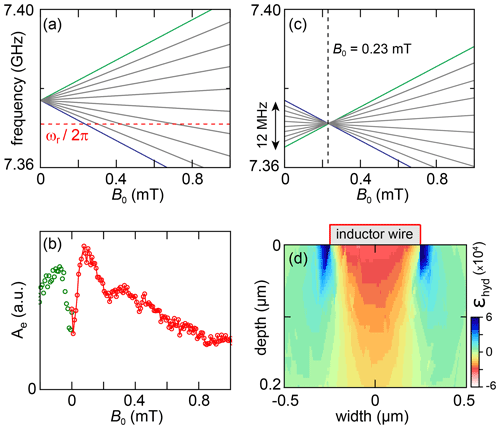Figure 8(a) EPR-allowed transitions of a bismuth donor in silicon for $\mathrm{0}<{B}_{\mathrm{0}}<\mathrm{1}$ mT. The dashed red line denotes the resonator frequency ωr. The spectrum is for an unstrained donor for which the frequency at B0=0 is 5ABi∕(2π). (b) Echo-detected field sweep. The echo integral Ae is plotted versus B0. (c) Frequency of all 18 bismuth donor transitions that may contribute to the echo signal at a given field (here, B0=0.23 mT). This is made possible by the strain-induced spread in ABi between different donors. (d) Hydrostatic component of strain in silicon simulated using COMSOL.

Download

### 5.2.2 Two-pulse ESEEM

Two-pulse echoes are measured with the pulse sequence shown in Fig. 1, which consists of a square π∕2X pulse of duration 50 ns followed by a square πY pulse of duration 100 ns after a delay τ. Note that due to the donor spatial location in a shallow layer below the surface and to the strain shifting of their Larmor frequency , the Rabi frequency is more homogeneous than in the erbium-doped sample, and Rabi rotations with a well-defined angle can be applied . To increase the signal-to-noise ratio, a CPMG sequence of 198 π pulses separated by 10µs are used following the echo sequence . The curves are repeated 20 times, with a delay of 2 s in between to enable spin relaxation of the donors. All the resulting echoes are then averaged. Phase cycling is performed by alternating sequences with opposite phases for the π∕2 pulses and subtracting the resulting echoes. The data are obtained in the low-Q configuration (see Sect. 4).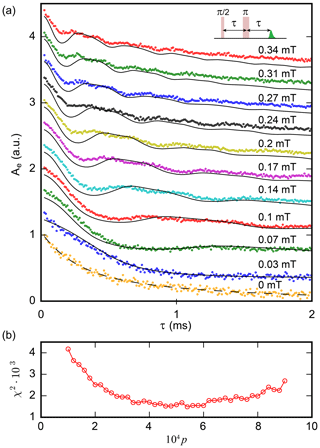Figure 9Two-pulse ESEEM of Bi:Si donors. (a) Echo integral Ae versus inter-pulse delay τ for a two-pulse echo sequence for varying magnetic field B0. Dots indicate experimental data; lines indicate results of the model (see text), assuming a concentration in 29Si of $p=\mathrm{4.4}×{\mathrm{10}}^{-\mathrm{4}}$. The curves are vertically shifted for clarity. (b) Fit residue χ2 for different 29Si relative abundance p. The best fit is obtained for $p=\mathrm{4.4}±\mathrm{1}×{\mathrm{10}}^{-\mathrm{4}}$, in agreement with the specified value.

Download

Figure 9 shows the integral of the averaged echoes Ae(τ) as a function of τ for various values of B0 . At a non-zero field, Ae(τ) shows B0-dependent oscillations on top of an exponential decay with time constant T2=2.6 ms. Similar decay times were measured on the same chip with another resonator , and are attributed to a combination of donor–donor dipolar interactions and magnetic noise from defects at the sample surface.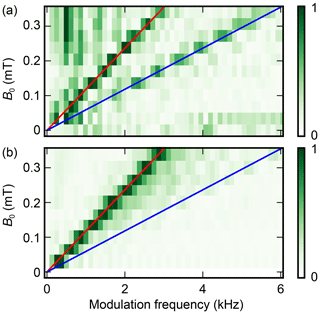Figure 10Amplitude of the Fourier transform of the experimental (a) and theoretical (b) two-pulse Bi:Si donor ESEEM data.

Download

In the subsequent discussion, we concentrate on the ESEEM pattern. To analyze the data, each curve was divided by a constant exponential decay with 2.6 ms time constant, mirrored at t=0, and Fourier transformed (see Fig. 10). Only two peaks are observed. Their frequencies vary linearly with B0, and are found to be approximately 8 and 16 kHz mT−1. This is in good agreement with the gyromagnetic ratio of 29Si (8.46 kHz mT−1); the presence of the second peak at twice this value is expected as explained in Sect. 2 for the two-pulse ESEEM in the weak-coupling limit. The oscillation amplitude goes down with B0, again as expected from the model put forward in Sect. 2.

A rough estimate of the number of donors contributing to the measurements shown in Fig. 9 can be obtained by comparison with . Given the nearly identical resonator geometry, and assuming identical strain broadening in both samples, the ratio of the number of donors involved in both measurements is simply given by the ratio of resonator bandwidths. For the low-Q configuration, such as the two-pulse echo of Fig. 9, this corresponds to $\simeq \mathrm{5}×{\mathrm{10}}^{\mathrm{3}}$ dopants; in the high-Q configuration (see the three- and five-pulse data in the next paragraph), this number is reduced to $\simeq \mathrm{5}×{\mathrm{10}}^{\mathrm{2}}$ dopants.

### 5.2.3 Three- and five-pulse ESEEM

The spectral resolution provided by the measurement protocol is limited because of the finite electron coherence time T2. As discussed in Sect. 2.3, this can be overcome by three- or five-pulse ESEEM.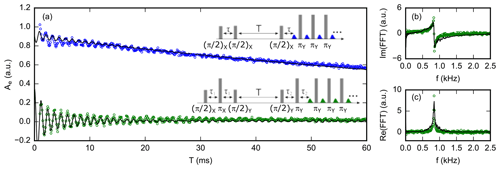Figure 11(a) Three-pulse (blue circles) and five-pulse (green circles) ESEEM signals of Bi:Si donors at B0=0.1 mT. Black lines are simulations assuming a 29Si concentration of $p=\mathrm{4.4}×{\mathrm{10}}^{-\mathrm{4}}$. (b) Imaginary and (c) real part of the Fourier transform of the five-pulse ESEEM data. The spectrum only contains a peak at 850 Hz, which is the 29Si nuclei Larmor frequency at this field.

Download

We measure three- and five-pulse ESEEM with the pulse sequence shown in Fig. 11. The high-Q configuration is chosen for which T1=120 ms is measured (see the Supplement); shaped pulses generate an intra-cavity field in the form of a rectangular pulse of 1µs duration with sharp rise and fall despite the high resonator quality factor. The data are acquired at B0=0.1 mT, so that ${\mathit{\omega }}_{I}/\mathrm{2}\mathit{\pi }\simeq \mathrm{850}$ Hz. The first blind spot for three-pulse ESEEM is thus at $\mathrm{2}\mathit{\pi }/{\mathit{\omega }}_{I}=\mathrm{1.2}$ ms; we chose τ=290µs for the three-pulse echo, and ${\mathit{\tau }}_{\mathrm{1}}={\mathit{\tau }}_{\mathrm{2}}=\mathrm{290}$µs for the five-pulse sequence. A sequence of 19 CPMG π pulses, separated by 50µs, was used to enhance the signal-to-noise ratio. The sequences were repeated after a fixed waiting time of 100 ms between the last π pulse of one sequence and the first π∕2 pulse of the following, to enable spin relaxation. Phase cycling is used to suppress unwanted echoes (see the Supplement for the schemes; ). Each point is averaged over 2.5×104 sequences, with a total acquisition time of 2 weeks for each curve .

The results are shown in Fig. 11, together with their fast Fourier transform . Both the three-pulse ESEEM (3PE) and five-pulse ESEEM (5PE) curves show oscillations that last 1 order of magnitude longer than the electron spin T2 (up to 20 ms), enabling higher spectral resolution of the ESEEM signal. The 5PE curve has a higher oscillation amplitude than the 3PE by a factor of 2–3, as expected. The decay of the oscillations occurs in ∼10 ms, 1 order of magnitude faster than the stimulated echo amplitude (see the 3PE curve), suggesting that it is an intrinsic feature of the ESEEM signal, as discussed below.

The spectrum shows only one peak at the 29Si frequency. This is consistent with the expression provided in Sect. 2 and the Supplement for the three- and five-pulse ESEEM, in which the terms oscillating at the sum and difference frequency are absent in contrast to the two-pulse ESEEM. The peak width is ≃100 Hz, which indicates that the nuclei contributing to the ESEEM signal have hyperfine coupling strengths A, B of at most 100 Hz. Neglecting the contact interaction term, this corresponds to 29Si nuclei that are located at least ∼5 nm away from the donor spin.

The measured ESEEM spectrum of the bismuth donor sample qualitatively differs from the erbium sample, since it only contains a peak at the unperturbed silicon nuclei Larmor frequency (and at twice this frequency for the two-pulse ESEEM), instead of the many peaks observed in Fig. 7 indicating nuclear-spin contribution with vastly different hyperfine strengths. This can be qualitatively understood by examining Eq. (8). Defining Nl as the number of lattice sites with approximately the same hyperfine parameters Al, Bl and modulation frequency ${\mathit{\omega }}_{↓/↑,l}$, the component at ${\mathit{\omega }}_{↓/↑,l}$ is visible in the spectrum if Nlklp∼1, which can only be achieved if Nlp∼1. In the case of erbium, p=0.144 so that even the sites closest to the ion (for which Nl is of order unity) may satisfy this condition for well-chosen B0. In the bismuth donor sample where $p=\mathrm{4.4}×{\mathrm{10}}^{-\mathrm{4}}$, this condition can only be met for Nl∼103, and therefore for crystal sites l that are far from the donor, for which the hyperfine coupling is small, so that ${\mathit{\omega }}_{↓/↑,l}\simeq {\mathit{\omega }}_{I}$. This is confirmed by the more quantitative modeling below.

### 5.2.4 Comparison with the model

As explained above, the measured echo signal results from the contribution of all 18 Bi:Si transitions because of strain broadening. To model the data, we therefore apply the fictitious spin-1∕2 model to each transition, and sum the resulting echo amplitudes weighted by their relative contribution, which we determine using numerical simulations described in the Supplement.

Moreover, as discussed in Sect. 3.2, and in contrast to the erbium case, the fictitious spin model for a given transition needs to be validated in the low-B0 regime because the energy difference between neighboring hyperfine levels of the bismuth donor manifold (${E}_{m}^{±}-{E}_{m-\mathrm{1}}^{±}\right)/h\simeq \mathrm{0.3}$ MHz for B0=0.1 mT is comparable to or even lower than the hyperfine coupling to some 29Si nuclei. In that case, the hyperfine interaction induces significant mixing between the bismuth donor and the 29Si eigenstates, and we should describe the coupled “electron spin S0+209Bi nuclear spin I0+29Si nuclear spin I” system as a single 40-level quantum system.

This study is described in Sect. S5 for a 29Si with strong hyperfine coupling (≥200 kHz). The state mixing makes many transitions EPR allowed, and the interference between these transitions causes fast oscillations in the spin-echo signal, as seen in Fig. S7 in the Supplement. The frequencies of these oscillations depend greatly on the local Overhauser field on the donor electron spin. Since the latter has a large inhomogeneous broadening (∼0.5 MHz), the ensemble average leads to a rapid decay of the signal (<1µs). Given the 29Si concentration, about 10 % of the donors have one or more 29Si with coupling >300 kHz in the proximity, which therefore leads to a rapid decay of the total echo signal within ∼1µs by about 10 %. In the experimental data, this fast decay is not visible because the echo signal is measured at longer times, and therefore the ESEEM signals presented in Fig. S5 are those from 29Si with couplings <200 kHz.

As for spins with a coupling strength between 20 and 200 kHz, they lead to ESEEM amplitude much less than 1 %, as shown in Figs. S7–S9. For nuclear spins with a hyperfine coupling <100 kHz, the fictitious spin model produces results with negligible errors of the modulation frequencies from the exact solution (Figs. S5 and S6). Furthermore, the systematic numerical studies (Figs. S9) show that a nearby Si nuclear spin with coupling <100 kHz has little effect on the ESEEM due to other distant nuclear spins.

Considering these different contributions of Si nuclear spins of different hyperfine couplings, as discussed in the paragraph above and in more details in the Supplement, we apply the fictitious spin-1∕2 model to each EPR-allowed transition of the bismuth donor manifold, considering only Si nuclear spins that have a hyperfine coupling weaker than a certain cut-off which we choose as 20 kHz, and discarding all the others.

For each transition, we compute the hyperfine parameters that enter the fictitious spin-1∕2 model for all sites of the silicon lattice. We then generate a large number of random configurations of nuclear spins. We compute the corresponding two-, three-, or five-pulse ESEEM signal using the analytical formulas of Sect. 2.4 after discarding all nuclei whose hyperfine coupling is larger than 20 kHz. We average the signal for one configuration over all bismuth donor transitions using the weights determined by simulation and then average the results over all the configurations computed. In this way, we obtain the curves shown in Fig. 9.

We use the two-pulse echo dataset to determine the most likely sample concentration in 29Si, using p as a fitting parameter. As seen in Fig. 9b, the best fit is obtained for $p=\mathrm{4.4}±\mathrm{1}×{\mathrm{10}}^{-\mathrm{4}}$, which is compatible with the specified $\mathrm{5}×{\mathrm{10}}^{-\mathrm{4}}$. The agreement is satisfactory but not perfect, as seen, for instance, in the amplitude of the short-time ESEEM oscillations which are lower in the measurements than in the simulations, particularly at larger field. Also, the peak at 2ωI is notably broader and has a lower amplitude than in the experiment.

For the fitted value of p, the three- and five-pulse theoretical signals are also computed and found to be in overall agreement with the data, even though the decay of the ESEEM signal predicted by the model is faster than in the experiment and correspondingly the predicted ESEEM spectrum broader than the data.

6 Discussion and conclusion

We have reported two-, three-, and five-pulse ESEEM measurements using a quantum-limited EPR spectrometer on two model systems: erbium ions in a CaWO4 matrix and bismuth donors in silicon. Whereas the erbium measurements are done in a commonly used regime of high field, the bismuth donor measurements are performed in an unusual regime of low nuclear-spin density, low hyperfine coupling, and almost zero magnetic field. Good agreement is found with the simplest analytical ESEEM models.

Having demonstrated that ESEEM is feasible in a millikelvin quantum-limited EPR spectrometer setup on two model spin systems, it is worth speculating in broader terms about its potential for real-world hyperfine spectroscopy. First, high magnetic fields are desirable for a better spectral resolution. Superconducting resonators in Nb, niobium nitride (NbN), or niobium titanium nitride (NbTiN) can retain a high quality factor up to ∼1 T , so that quantum-limited EPR spectroscopy in the Q band can in principle be envisioned. Resonator bandwidths larger than demonstrated here are also desirable. Given, increasing κ in the Purcell regime leads to longer relaxation times T1, this should be done with care. One option is to increase also the coupling constant g by further reduction of the resonator mode volume . Interestingly, this provides another motivation to apply higher magnetic fields, since g is proportional to ωr. Overall, a resonator at ${\mathit{\omega }}_{\mathrm{r}}/\mathrm{2}\mathit{\pi }\simeq \mathrm{30}$ GHz, in a magnetic field B0≃1 T, and with a $\mathit{\kappa }/\mathrm{2}\mathit{\pi }\sim \mathrm{10}$ MHz bandwidth seems within reach, while keeping the Purcell T1 well below 1 s. Such a high-bandwidth, high-sensitivity EPR spectrometer would be ideally suited for studying surface defects. One potential concern, however, is the power-handling capability of the resonator, as the kinetic inductance causes a non-linear response at high power.

Code and data availability

All code and data necessary for generating Figs. 6–11 can be found at https://doi.org/10.7910/DVN/ZJ2EEX . The analysis and plotting code is written in Python (.py) and Igor (.pxp). These files are sorted according to figure number, with the relevant files for each figure compressed into a single 7zip file (.7z).

Supplement

The supplement related to this article is available online at: https://doi.org/10.5194/mr-1-315-2020-supplement.

Author contributions

SP, MR, MLD, AD, and PB planned and designed the experiment. ZZ and PG prepared the Er:CaWO4 crystal. JM prepared and provided the bismuth-donor-implanted silicon sample. SP, MR, and MLD fabricated the devices, set up the experiment, and acquired the data. SP, GLZ, MR, VR, MLD, BA, AD, RBL, TC, PG, and PB worked on the data analysis. The project was supervised by RBL and PB. All authors contributed to manuscript preparation.

Competing interests

The authors declare that they have no conflict of interest.

Acknowledgements

We thank Pascal Sénat, Dominique Duet, and Jean-Claude Tack for the technical support and are grateful for fruitful discussions within the Quantronics group. We acknowledge IARPA and Lincoln Labs for providing a Josephson traveling-wave parametric amplifier used in some of the measurements. We acknowledge support of the European Research Council under the European Community's Seventh Framework Programme (FP7/2007-2013) and under the European Union's Horizon 2020 research and innovation programme of the Agence Nationale de la Recherche under the Chaire Industrielle NASNIQ supported by Atos, the QIPSE project (Hong Kong RGC – French ANR Joint Scheme Fund Project A-CUHK403/15), and the MIRESPIN project, and of Région Île-de-France Domaine d'Interet Majeur SIRTEQ under grant REIMIC. Miloš Rančić acknowledges a Marie Curie Individual Grant of the European Union. Andrin Doll acknowledges a SNSF mobility fellowship.

Financial support

This research has been supported by the European Commission through the H2020 Marie Skłodowska-Curie Action (SMERC (grant no. 792727)), the Seventh Framework Programme (FP7/2007-2013) (CIRQUSS (grant agreement no. 615767)), and the Horizon 2020 Framework Programme, H2020 Excellent Science (LOQO-MOTIONS (grant agreement no. 771493)); the Agence Nationale de la Recherche (grants nos. ANR-17-CHIN-0001 and A-CUHK403/15); the Domaine d'Intérêt Majeur Science et Ingénierie en Région Île-de-France pour les Technologies (SIRTEQ (grant no. REIMIC)), and the Swiss National Science Foundation (SNSF) (grant no. 177732).

Review statement

This paper was edited by Robert Bittl and reviewed by Graham Smith and Edgar Groenen.

References

Abe, E., Tyryshkin, A. M., Tojo, S., Morton, J. J. L., Witzel, W. M., Fujimoto, A., Ager, J. W., Haller, E. E., Isoya, J., Lyon, S. A., Thewalt, M. L. W., and Itoh, K. M.: Electron spin coherence of phosphorus donors in silicon: Effect of environmental nuclei, Phys. Rev. B, 82, 121201, https://doi.org/10.1103/PhysRevB.82.121201, 2010. a

Abragam, A. and Bleaney, B.: Electron Paramagnetic Resonance of Transition Ions, OUP Oxford, UK, google-Books-ID: ASNoAgAAQBAJ, ISBN 987-0-19-965152-8, 13 pp., 2012. a

Antipin, A., Katyshev, A., Kurkin, I., and Shekun, L.: Paramagnetic resonance and spin-lattice relaxation of Er3+ and Tb3+ ions in CaWO4 crystal lattice, Sov. Phys. Solid State, 10, 468–474, 1968. a

Artzi, Y., Twig, Y., and Blank, A.: Induction-detection electron spin resonance with spin sensitivity of a few tens of spins, Appl. Phys. Lett., 106, 084104, https://doi.org/10.1063/1.4913806, 2015. a

Baumann, S., Paul, W., Choi, T., Lutz, C. P., Ardavan, A., and Heinrich, A. J.: Electron paramagnetic resonance of individual atoms on a surface, Science, 350, 417–420, https://doi.org/10.1126/science.aac8703, 2015. a

Benningshof, O. W. B., Mohebbi, H. R., Taminiau, I. A. J., Miao, G. X., and Cory, D. G.: Superconducting microstrip resonator for pulsed ESR of thin films, J. Magn. Reson., 230, 84–87, https://doi.org/10.1016/j.jmr.2013.01.010, 2013. a

Bienfait, A., Pla, J., Kubo, Y., Stern, M., Zhou, X., Lo, C.-C., Weis, C., Schenkel, T., Thewalt, M., Vion, D., Esteve, D., Julsgaard, B., Moelmer, K., Morton, J., and Bertet, P.: Reaching the quantum limit of sensitivity in electron spin resonance, Nat. Nanotechnol., 11, 253–257, https://doi.org/10.1038/nnano.2015.282, 2015. a, b, c, d, e, f, g

Bienfait, A., Pla, J., Kubo, Y., Zhou, X., Stern, M., Lo, C.-C., Weis, C., Schenkel, T., Vion, D., Esteve, D., Morton, J., and Bertet, P.: Controlling Spin Relaxation with a Cavity, Nature, 531, 74–77, https://doi.org/10.1038/nature16944, 2016. a

Byrd, R., Lu, P., Nocedal, J., and Zhu, C.: A Limited Memory Algorithm for Bound Constrained Optimization, SIAM J. Sci. Comput., 16, 1190–1208, https://doi.org/10.1137/0916069, 1995. a

Car, B., Veissier, L., Louchet-Chauvet, A., Le Gouët, J.-L., and Chanelière, T.: Selective Optical Addressing of Nuclear Spins through Superhyperfine Interaction in Rare-Earth Doped Solids, Phys. Rev. Lett., 120, 197401, https://doi.org/10.1103/PhysRevLett.120.197401, 2018. a

Childress, L., Dutt, M. V. G., Taylor, J. M., Zibrov, A. S., Jelezko, F., Wrachtrup, J., Hemmer, P. R., and Lukin, M. D.: Coherent Dynamics of Coupled Electron and Nuclear Spin Qubits in Diamond, Science, 314, 281–285, https://doi.org/10.1126/science.1131871, 2006. a

Day, P. K., LeDuc, H. G., Mazin, B. A., Vayonakis, A., and Zmuidzinas, J.: A broadband superconducting detector suitable for use in large arrays, Nature, 425, 817–821, https://doi.org/10.1038/nature02037, 2003. a

Eichler, C., Sigillito, A. J., Lyon, S. A., and Petta, J. R.: Electron Spin Resonance at the Level of 104 Spins Using Low Impedance Superconducting Resonators, Phys. Rev. Lett., 118, 037701, https://doi.org/10.1103/PhysRevLett.118.037701, 2017. a, b, c

Elzerman, J. M., Hanson, R., Beveren, L. H. W. v., Witkamp, B., Vandersypen, L. M. K., and Kouwenhoven, L. P.: Single-shot read-out of an individual electron spin in a quantum dot, Nature, 430, 431–435, https://doi.org/10.1038/nature02693, 2004. a

Feher, G.: Electron Spin Resonance Experiments on Donors in Silicon. I. Electronic Structure of Donors by the Electron Nuclear Double Resonance Technique, Phys. Rev., 114, 1219–1244, https://doi.org/10.1103/PhysRev.114.1219, 1959. a

Graaf, S. E. d., Danilov, A. V., Adamyan, A., Bauch, T., and Kubatkin, S. E.: Magnetic field resilient superconducting fractal resonators for coupling to free spins, J. Appl. Phys., 112, 123905, https://doi.org/10.1063/1.4769208, 2012. a

Guillot-Noël, O., Vezin, H., Goldner, P., Beaudoux, F., Vincent, J., Lejay, J., and Lorgeré, I.: Direct observation of rare-earth-host interactions in Er:Y2SiO5, Phys. Rev. B, 76, 180408, https://doi.org/10.1103/PhysRevB.76.180408, 2007. a

Hale, E. B. and Mieher, R. L.: Shallow Donor Electrons in Silicon. II. Considerations Regarding the Fermi Contact Interactions, Phys. Rev., 184, 751–759, https://doi.org/10.1103/physrev.184.751, 1969. a

Jelezko, F., Gaebel, T., Popa, I., Gruber, A., and Wrachtrup, J.: Observation of Coherent Oscillations in a Single Electron Spin, Phys. Rev. Lett., 92, 076401, https://doi.org/10.1103/PhysRevLett.92.076401, 2004. a

Kasumaj, B. and Stoll, S.: 5- and 6-pulse electron spin echo envelope modulation (ESEEM) of multi-nuclear spin systems, J. Magn. Reson., 190, 233–247, https://doi.org/10.1016/j.jmr.2007.11.001, 2008. a, b, c

Kohn, W. and Luttinger, J. M.: Theory of Donor States in Silicon, Physical Review, 98, 915–922, https://doi.org/10.1103/PhysRev.98.915, 1955. a

Kubo, Y., Ong, F. R., Bertet, P., Vion, D., Jacques, V., Zheng, D., Dréau, A., Roch, J.-F., Auffeves, A., Jelezko, F., Wrachtrup, J., Barthe, M. F., Bergonzo, P., and Esteve, D.: Strong Coupling of a Spin Ensemble to a Superconducting Resonator, Phys. Rev. Lett., 105, 140502, https://doi.org/10.1103/PhysRevLett.105.140502, 2010. a

Macklin, C., O’Brien, K., Hover, D., Schwartz, M. E., Bolkhovsky, V., Zhang, X., Oliver, W. D., and Siddiqi, I.: A near–quantum-limited Josephson traveling-wave parametric amplifier, Science, 350, 307–310, https://doi.org/10.1126/science.aaa8525, 2015. a

Mahashabde, S., Otto, E., Montemurro, D., de Graaf, S., Kubatkin, S., and Danilov, A.: Fast Tunable High-Q-Factor Superconducting Microwave Resonators, Phys. Rev. Applied 14, 044040, https://doi.org/10.1103/PhysRevApplied.14.044040, 2020. a

Mansir, J., Conti, P., Zeng, Z., Pla, J., Bertet, P., Swift, M., Van de Walle, C., Thewalt, M., Sklenard, B., Niquet, Y., and Morton, J.: Linear Hyperfine Tuning of Donor Spins in Silicon Using Hydrostatic Strain, Phys. Rev. Lett., 120, 167701, https://doi.org/10.1103/PhysRevLett.120.167701, 2018. a

Mims, W. B.: Envelope Modulation in Spin-Echo Experiments, Phys. Rev. B, 5, 2409–2419, https://doi.org/10.1103/physrevb.5.2409, 1972. a

Mims, W. B., Nassau, K., and McGee, J. D.: Spectral Diffusion in Electron Resonance Lines, Phys. Rev., 123, 2059–2069, https://doi.org/10.1103/PhysRev.123.2059, 1961. a

Mims, W. B., Davis, J. L., and Peisach, J.: The exchange of hydrogen ions and of water molecules near the active site of cytochrome c, J. Magn. Reson., 86, 273–292, https://doi.org/10.1016/0022-2364(90)90260-G, 1990. a

Mohammady, M. H., Morley, G. W., and Monteiro, T. S.: Bismuth Qubits in Silicon: The Role of EPR Cancellation Resonances, Phys. Rev. Lett., 105, 067602, https://doi.org/10.1103/physrevlett.105.067602, 2010. a, b

Morello, A., Pla, J. J., Zwanenburg, F. A., Chan, K. W., Tan, K. Y., Huebl, H., Mottonen, M., Nugroho, C. D., Yang, C., van Donkelaar, J. A., Alves, A. D. C., Jamieson, D. N., Escott, C. C., Hollenberg, L. C. L., Clark, R. G., and Dzurak, A. S.: Single-shot readout of an electron spin in silicon, Nature, 467, 687–691, https://doi.org/10.1038/nature09392, 2010. a

Narkowicz, R., Suter, D., and Niemeyer, I.: Scaling of sensitivity and efficiency in planar microresonators for electron spin resonance, Review of Scientific Instruments, 79, 084702, https://doi.org/10.1063/1.2964926, 2008. a

Pla, J., Bienfait, A., Pica, G., Mansir, J., Mohiyaddin, F., Zeng, Z., Niquet, Y., Morello, A., Schenkel, T., Morton, J., and Bertet, P.: Strain-Induced Spin-Resonance Shifts in Silicon Devices, Phys. Rev. Appl., 9, 044014, https://doi.org/10.1103/PhysRevApplied.9.044014, 2018. a, b, c

Pla, J. J., Tan, K. Y., Dehollain, J. P., Lim, W. H., Morton, J. J. L., Jamieson, D. N., Dzurak, A. S., and Morello, A.: A single-atom electron spin qubit in silicon, Nature, 489, 541–545, https://doi.org/10.1038/nature11449, 2012. a

Probst, S., Rotzinger, H., Wünsch, S., Jung, P., Jerger, M., Siegel, M., Ustinov, A. V., and Bushev, P. A.: Anisotropic Rare-Earth Spin Ensemble Strongly Coupled to a Superconducting Resonator, Phys. Rev. Lett., 110, 157001, https://doi.org/10.1103/PhysRevLett.110.157001, 2013. a

Probst, S., Rotzinger, H., Ustinov, A. V., and Bushev, P. A.: Microwave multimode memory with an erbium spin ensemble, Phys. Rev. B, 92, 014421, https://doi.org/10.1103/PhysRevB.92.014421, 2015. a

Probst, S., Bienfait, A., Campagne-Ibarcq, P., Pla, J. J., Albanese, B., Barbosa, J. F. D. S., Schenkel, T., Vion, D., Esteve, D., Moelmer, K., Morton, J. J. L., Heeres, R., and Bertet, P.: Inductive-detection electron-spin resonance spectroscopy with 65 spins$/\sqrt{\mathrm{Hz}}$ sensitivity, Appl. Phys. Lett., 111, 202604, https://doi.org/10.1063/1.5002540, 2017. a, b, c, d, e, f, g, h, i, j

Probst, S., Ranjan, V., Ansel, Q., Heeres, R., Albanese, B., Albertinale, E., Vion, D., Esteve, D., Glaser, S. J., Sugny, D., and Bertet, P.: Shaped pulses for transient compensation in quantum-limited electron spin resonance spectroscopy, J. Magn. Reson., 303, 42–47, https://doi.org/10.1016/j.jmr.2019.04.008, 2019. a, b

Probst, S., Zhang, G., Rančić, M., Ranjan, V., Le Dantec, M., Zhang, Z., Albanese, B., Doll, A., Liu, R. B., Morton, J., Chanelière, T., Goldner, P., Vion, D., Esteve, D., and Bertet, P.: Replication Data for: Hyperfine spectroscopy in a quantum-limited spectrometer (2020), Harvard Dataverse, https://doi.org/10.7910/DVN/ZJ2EEX, 2020. a, b, c, d, e, f, g

Ranjan, V., Probst, S., Albanese, B., Doll, A., Jacquot, O., Flurin, E., Heeres, R., Vion, D., Esteve, D., Morton, J. J. L., and Bertet, P.: Pulsed electron spin resonance spectroscopy in the Purcell regime, J. Magn. Reson., 310, 106662, https://doi.org/10.1016/j.jmr.2019.106662, 2020a. a, b

Ranjan, V., Probst, S., Albanese, B., Schenkel, T., Vion, D., Esteve, D., Morton, J. J. L., and Bertet, P.: Electron spin resonance spectroscopy with femtoliter detection volume, Appl. Phys. Lett., 116, 184002, https://doi.org/10.1063/5.0004322, 2020b. a, b

Rowan, L. G., Hahn, E. L., and Mims, W. B.: Electron-Spin-Echo Envelope Modulation, Phys. Rev., 137, A61–A71, https://doi.org/10.1103/physrev.137.a61, 1965. a, b, c

Rugar, D., Budakian, R., Mamin, H., and Chui, B.: Single spin detection by magnetic resonance force microscopy, Nature, 430, 329–332, https://doi.org/10.1038/nature02658, 2004. a

Samkharadze, N., Bruno, A., Scarlino, P., Zheng, G., DiVincenzo, D., DiCarlo, L., and Vandersypen, L.: High-Kinetic-Inductance Superconducting Nanowire Resonators for Circuit QED in a Magnetic Field, Phys. Rev. Appl., 5, 044004, https://doi.org/10.1103/PhysRevApplied.5.044004, 2016.  a

Schweiger, A. and Jeschke, G.: Principles of pulse electron paramagnetic resonance, 1st edn., Oxford University Press, Oxford, UK, ISBN: 0 19 850634 1, 2001. a, b, c, d, e, f, g, h, i, j

Sidabras, J. W., Duan, J., Winkler, M., Happe, T., Hussein, R., Zouni, A., Suter, D., Schnegg, A., Lubitz, W., and Reijerse, E. J.: Extending electron paramagnetic resonance to nanoliter volume protein single crystals using a self-resonant microhelix, Science Advances, 5, eaay1394, https://doi.org/10.1126/sciadv.aay1394, 2019. a

Sigillito, A. J., Malissa, H., Tyryshkin, A. M., Riemann, H., Abrosimov, N. V., Becker, P., Pohl, H.-J., Thewalt, M. L. W., Itoh, K. M., Morton, J. J. L., Houck, A. A., Schuster, D. I., and Lyon, S. A.: Fast, low-power manipulation of spin ensembles in superconducting microresonators, Appl. Phys. Lett., 104, 222407, https://doi.org/10.1063/1.4881613, 2014. a

Sigillito, A., Tyryshkin, A., Schenkel, T., Houck, A. A., and Lyon, S. A.: All-electric control of donor nuclear spin qubits in silicon, Nature Nanotech, 12, 958–962, https://doi.org/10.1038/nnano.2017.154, 2017. a

Song, L., Liu, Z., Kaur, P., Esquiaqui, J. M., Hunter, R. I., Hill, S., Smith, G. M., and Fanucci, G. E.: Toward increased concentration sensitivity for continuous wave EPR investigations of spin-labeled biological macromolecules at high fields, J. Magn. Reson., 265, 188–196, https://doi.org/10.1016/j.jmr.2016.02.007, 2016. a

Veldhorst, M., Hwang, J. C. C., Yang, C. H., Leenstra, A. W., de Ronde, B., Dehollain, J. P., Muhonen, J. T., Hudson, F. E., Itoh, K. M., Morello, A., and Dzurak, A. S.: An addressable quantum dot qubit with fault-tolerant control-fidelity, Nat. Nanotechnol., 9, 981–985, https://doi.org/10.1038/nnano.2014.216, 2014. a

Wallace, W. J. and Silsbee, R. H.: Microstrip resonators for electron‐spin resonance, Review of Scientific Instruments, 62, 1754–1766, https://doi.org/10.1063/1.1142418, 1991. a

Witzel, W. M., Hu, X., and Das Sarma, S.: Decoherence induced by anisotropic hyperfine interaction in Si spin qubits, Phys. Rev. B, 76, 035212, https://doi.org/10.1103/PhysRevB.76.035212, 2007. a

Wolfowicz, G., Tyryshkin, A. M., George, R. E., Riemann, H., Abrosimov, N. V., Becker, P., Pohl, H.-J., Thewalt, M. L. W., Lyon, S. A., and Morton, J. J. L.: Atomic clock transitions in silicon-based spin qubits, Nat. Nanotechnol., 8, 561–564, https://doi.org/10.1038/nnano.2013.117, 2013. a

Wrachtrup, J., Von Borczyskowski, C., Bernard, J., Orritt, M., and Brown, R.: Optical detection of magnetic resonance in a single molecule, Nature, 363, 244–245, https://doi.org/10.1038/363244a0, 1993. a

Zhou, X., Schmitt, V., Bertet, P., Vion, D., Wustmann, W., Shumeiko, V., and Esteve, D.: High-gain weakly nonlinear flux-modulated Josephson parametric amplifier using a SQUID array, Phys. Rev. B, 89, 214517, https://doi.org/10.1103/PhysRevB.89.214517, 2014. a